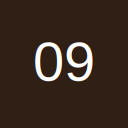# find and change worksheet name

• I've got macro written for "Sheet1", with "Sheet1" through the code.
I want to use this macro on worksheets with different from "Sheet1" names.
Every time I will use the macro, it will work only on currently open active sheet.
How can I change macro code to work on current macro, even it's name is not "Sheet1" ?

• Re: find and change worksheet name

Can you post the current code?

You can reference the active sheet like this.

Code
``Msgbox ActiveSheet.Name``

Boo!:yikes:

• Re: find and change worksheet name

Thank you for suggestion. I used your idea to capture sheet name , my whole macro is below. It's working now. I use variable "n" instead of sheet name. May be you think it can be improved somehow ?
For your info: this macro is printing labels from worksheet in particular format.
[vba]
Sub PrintLabels()
Dim x As Long
Dim y As Long
Dim z As Integer
Dim i As Integer
'for sheetname
Dim n As String

n = ActiveSheet.Name
Sheets(ActiveSheet.Name).Activate
x = InputBox("Enter the beginning row:", "Starting Row")
If Not IsNumeric(x) Or Not x > 1 Or Not x = Int(x) Then x = 2
y = InputBox("Enter the ending row:", "Finishing Row")
If Not IsNumeric(y) Or y < x Or Not y = Int(y) Then y = x
z = InputBox("Enter the number of copies to print:", "Copies to Print", 1)
If Not IsNumeric(z) Or z < 0 Or Not z = Int(z) Then z = 1
MsgBox "Ready to print " & z & " copies of rows " & x & " to " & y & "."

With Application
.ScreenUpdating = False
End With

On Error Resume Next
Sheets("Label").Delete
On Error GoTo 0
With ActiveSheet
.Name = "Label"
.Range("a1") = "Batch:"
.Range("a2") = "Date:"
.Range("a3") = "QTY:"
'.Range("a5") = "Company:"
.Cells.Font.Size = 36
.Range("a4").Font.Size = 80
.Range("a5").Font.Size = 60
.Range("b1").Font.Size = 50
.Range("b3").Font.Size = 50
.Range("a4").Font.Name = "Zebra Scalable"
.Range("a4").Font.Bold = True
.Range("a5").Font.Name = "Zebra Scalable"
.Range("b1:b5").Font.Name = "Zebra Scalable"
.Range("b2").NumberFormat = "m/d/yyyy"
With .PageSetup
.PrintArea = "\$A\$1:\$B\$5"
'.LeftMargin = Application.InchesToPoints(0.25)
'.RightMargin = Application.InchesToPoints(0.25)
'.TopMargin = Application.InchesToPoints(0)
'.BottomMargin = Application.InchesToPoints(0)
'.Orientation = xlLandscape
.LeftFooter = ""
.CenterFooter = ""
.RightFooter = ""
.LeftMargin = Application.InchesToPoints(0.25)
.RightMargin = Application.InchesToPoints(0.25)
.TopMargin = Application.InchesToPoints(0)
.BottomMargin = Application.InchesToPoints(0)
.FooterMargin = Application.InchesToPoints(0.5)
.PrintGridlines = False
.CenterHorizontally = False
.CenterVertically = False
.Orientation = xlLandscape
.Draft = False
' .PaperSize = 0
.FirstPageNumber = xlAutomatic
.Order = xlDownThenOver
.BlackAndWhite = False
.Zoom = False
.FitToPagesWide = 1
.FitToPagesTall = 1
.PrintErrors = xlPrintErrorsDisplayed
End With
'to wrap
Rows("4:4").Select
With Selection
.HorizontalAlignment = xlGeneral
.VerticalAlignment = xlBottom
.WrapText = True
.Orientation = 0
.IndentLevel = 0
.ShrinkToFit = False
.MergeCells = False
Selection.RowHeight = 260
' Selection.Font.Bold = True
End With
'end wrap
For i = x To y
.Range("b1") = Sheets(n).Cells(i, 1)
.Range("b2") = Sheets(n).Cells(i, 2)
.Range("a4") = Sheets(n).Cells(i, 4)
.Range("a5") = Sheets(n).Cells(i, 5)
.Columns("A:A").ColumnWidth = 90 'adjust as needed
.Columns("B:B").ColumnWidth = 28 'adjust as needed
' to choose printer
Application.Dialogs(xlDialogPrinterSetup).Show
ActiveWindow.SelectedSheets.PrintOut Copies:=z, ActivePrinter:= _
"Zebra S500/105S on COM2:", Collate:=True
Next i
End With
End Sub
[/vba]

## Participate now!

Don’t have an account yet? Register yourself now and be a part of our community!